# Basic Geometry : How to find the angle of clock hands

## Example Questions

2 Next →

### Example Question #11 : How To Find The Angle Of Clock Hands

Find the angle in degrees of the hands of a clock at 4:00.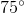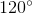Explanation:

To solve this question recall that a clock is a cicle and a circle is comprised of 360 degress.

To find how many degrees lie between each of the twelve numbers on the clock, divide 360 by 12.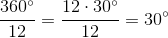.

From here, we will multiply 30 degrees by 4 to find the degree angle of for the hands of the clock at 4:00.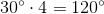Another approach is to simply divide 4 by 12 to determine what percentage of the circle the hands will cover, and then multiply that number by 360, which is the full degrees in a circle. Thus,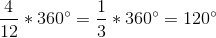.

### Example Question #11 : How To Find The Angle Of Clock Hands

A clock has the big hand at 12 o'clock and the little hand at 3 o'clock. What would be the angle for the configuration of these two hands?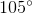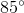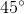Explanation:

With one hand ato'clock and the other ato'clock, the configuration encompasesof the complete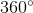of a full circle.

Therefore, we can determine the angle of the configuration as such: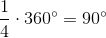### Example Question #11 : How To Find The Angle Of Clock Hands

The hour hand is on the 12 and the minute hand is on the 9. If I was to work clockwise, as in the way a clock goes, how many degrees is there from the hour hand to the minute hand?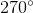Explanation:

There are 360 degrees in a circle. If you divide that by four then you get 90 degrees and if you divide 60 minutes by 4 you get 15 minutes. Therefore every 15 minutes on a clock represents 90 degrees. If I go clockwise from 12 there are 45 minutes or three lots of 15 minutes. If 1 lot of 15 minutes equals 90 degrees then,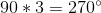### Example Question #11 : How To Find The Angle Of Clock Hands

If the hour hand is on the 12 and the minute hand is on the 3, what is the angle measure between them?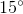Explanation:

It is important to recall that a clock is a circle and a circle is comprised of 360 degrees. Therefore, to calculate how many degrees are between the 12 and the 3 we need to set up a ratio.

The degree measure between each number is,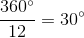.

Therefore, to find the degree measure for three numbers would be,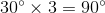.

If the hour hand is on the 12 and the minute hand is on the 3 it would create a right angle which is,.

### Example Question #11 : How To Find The Angle Of Clock Hands

If the hour hand is on the 12 and the minute hand is on the 6, what is the angle measure between them?Explanation:

It is important to recall that a clock is a circle and a circle is comprised of 360 degrees. Therefore, to calculate how many degrees are between the 12 and the 3 we need to set up a ratio.

The degree measure between each number is,.

Therefore, to find the degree measure between 6 numbers it would be,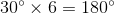.

If the hour hand is on the 12 and the minute hand is on the 6 it would create a straight line which is,.

### Example Question #12 : How To Find The Angle Of Clock Hands

At 12:45 AM, what is the angle formed between the minute and hour hands?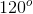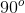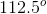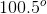Explanation:

The minute hand will be on the number 9, which would form a 90-degree angle with an hour hand pointing right at 12.

The hour hand has moved three-quarters, or 75% of the way from 12 to 1. Since the full 360 degrees of the circle represents 12 hours, the segment representing the hour between 12 and 1 is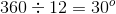.

75% of that is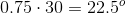.

The total angle is the 90 degrees between 9 and 12, and the additional 22.5 degrees, or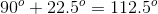### Example Question #12 : How To Find The Angle Of Clock Hands

Which of these times will have clock hands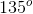apart?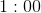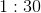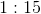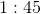Explanation:

We can automatically eliminate 1:00 and 1:15, since at those times the clock hands are less than 90 degrees apart. At 1:30 the hour hand is halfway between the 1 and the 2. Since there are 30 degrees between each number on the clock face, the hour hand is 30 + 15 = 45 degrees away from 12. The minute hand is on 6, which is 180 degrees from 12. The two hands form a 180 - 45 = 135 degree angle.

### Example Question #14 : How To Find The Angle Of Clock Hands

At 2:45 PM, how many degrees are between the minute and hour hands?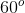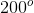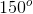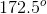Explanation:

At 2:45, the minute hand is on the 9, which is 90 degrees away from 12 to the left. The hour hand is three-quarters of the way between 2 and 3. Since there are 30 degrees between each number, this hand is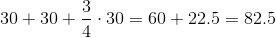degrees away from 12 to the right. The angle formed is 90 + 82.5 = 172.5 degrees.

### Example Question #15 : How To Find The Angle Of Clock Hands

True or false: At 3:30, the angle between the minute and hour hands is.

False

True

False

Explanation:

At 3:30, the minute hand is squarely on the 6, and the hour hand is halfway between the three and the four, as shown below: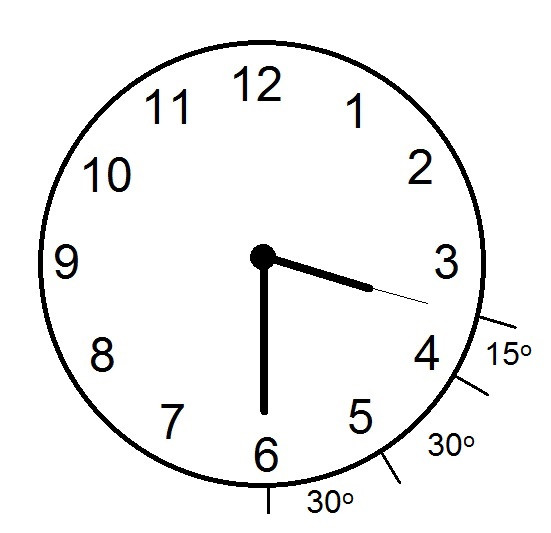As seen in the diagram, the degree measure from one hour mark to the next is one twelfth of, or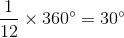. The degree measure from the six to halfway between the three and four is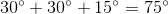- this is the angle the two hands form.

### Example Question #12 : How To Find The Angle Of Clock Hands

A clock is set at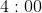. What is the angle in degrees between the minute hand and the hour hand?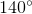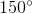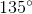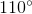Explanation:

The angle measure of one full revolution around the clock face is, since the clock face is circular. Looking at the numbers which indicate the time, one full revolution around the clock face also corresponds to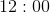. To find the angle measure between the minute hand and hour hand, consider the minute hand as the initial side of the angle and the hour side as the terminal side. The hour hand has rotated to theposition, so we can deduce the angle measureof the minute and hour hands by setting up and solving a proportion: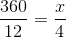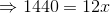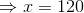Hence, the angle measure between the minute hand and hour hand of a clock set atis.

2 Next →

### All Basic Geometry Resources